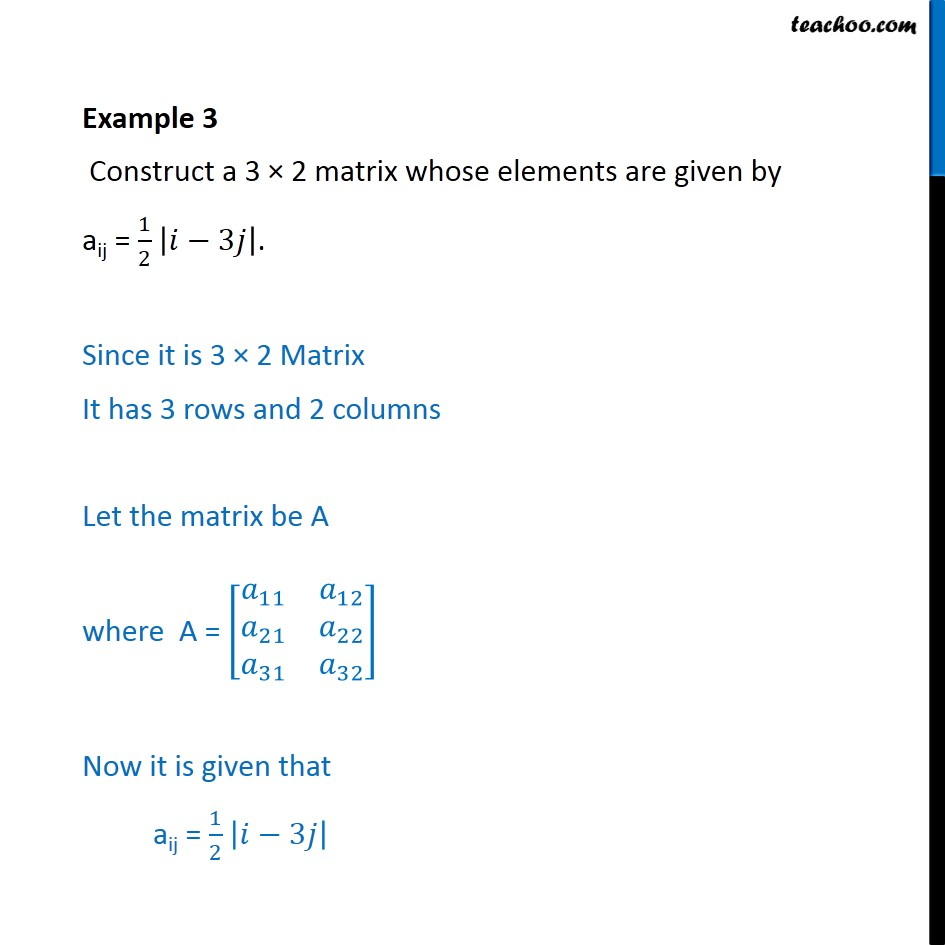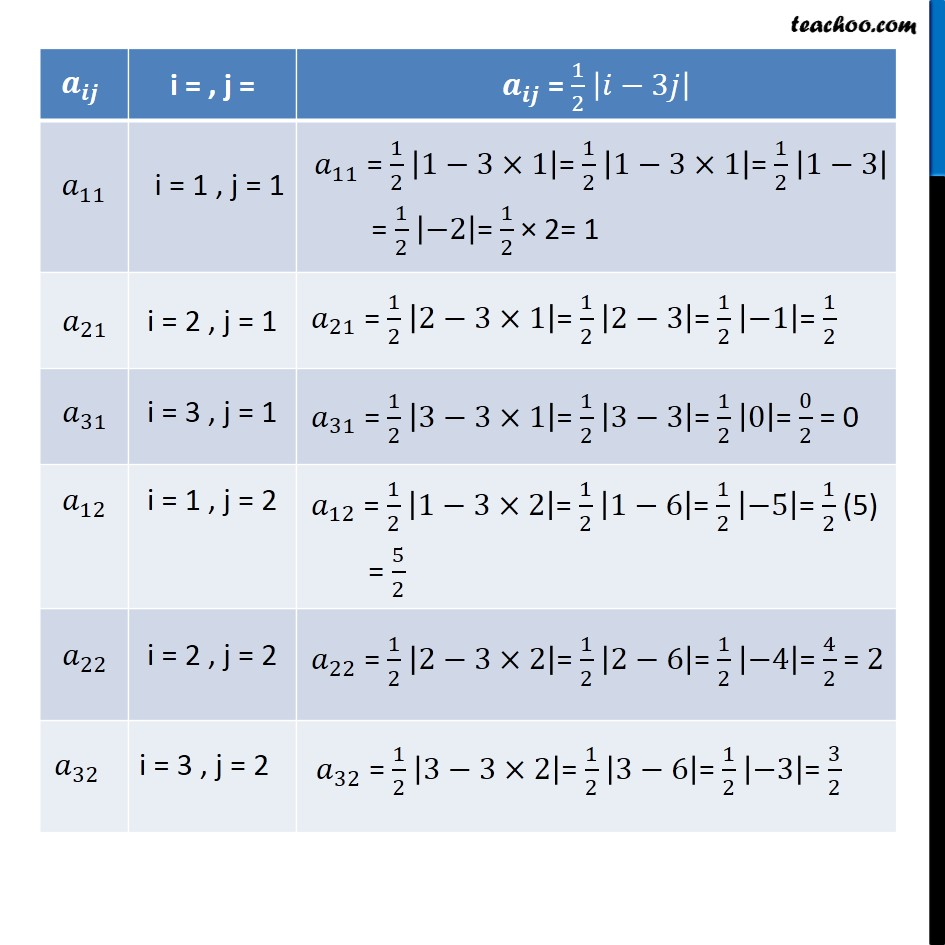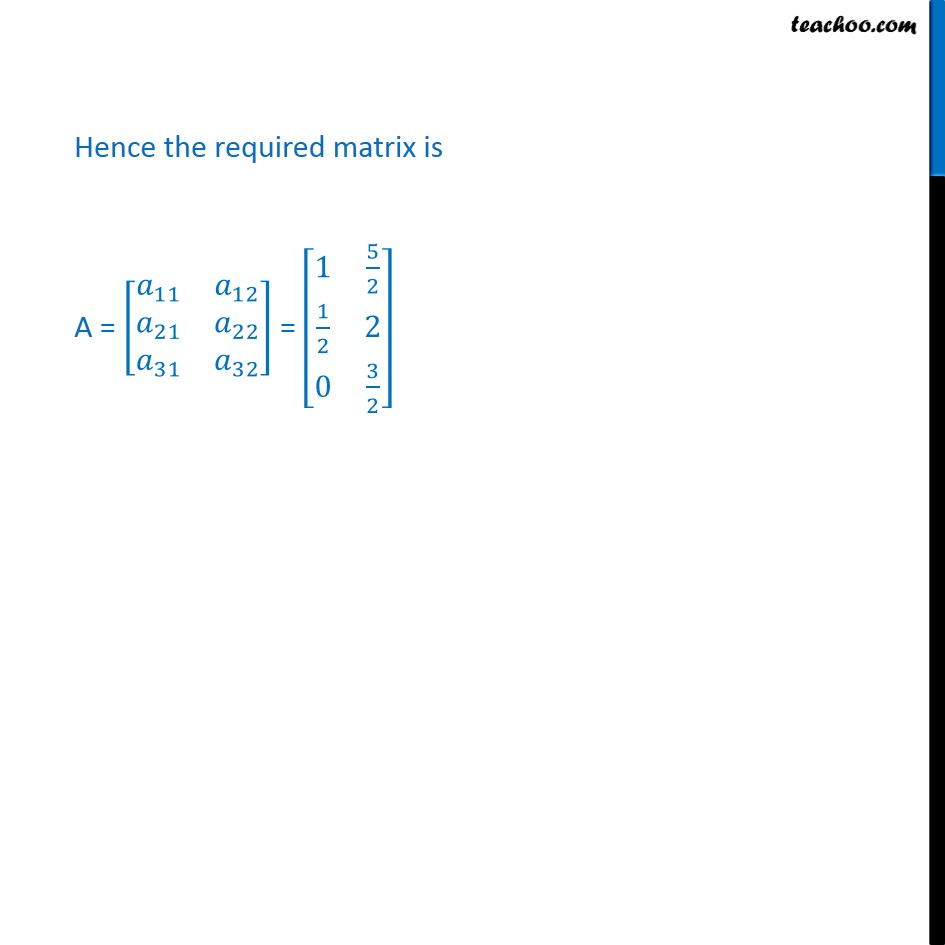1. Chapter 3 Class 12 Matrices (Term 1)
2. Concept wise
3. Formation and order of matrix

Transcript

Example 3 Construct a 3 × 2 matrix whose elements are given by aij = 1/2 |𝑖−3𝑗|. Since it is 3 × 2 Matrix It has 3 rows and 2 columns Let the matrix be A where A = [■8(𝑎_11&𝑎_12@𝑎_21&𝑎_22@𝑎_31&𝑎_32 )] Now it is given that aij = 1/2 |𝑖−3𝑗| Hence the required matrix is A = [■8(𝑎_11&𝑎_12@𝑎_21&𝑎_22@𝑎_31&𝑎_32 )] = [■8(1&5/2@1/2&2@0&3/2)]